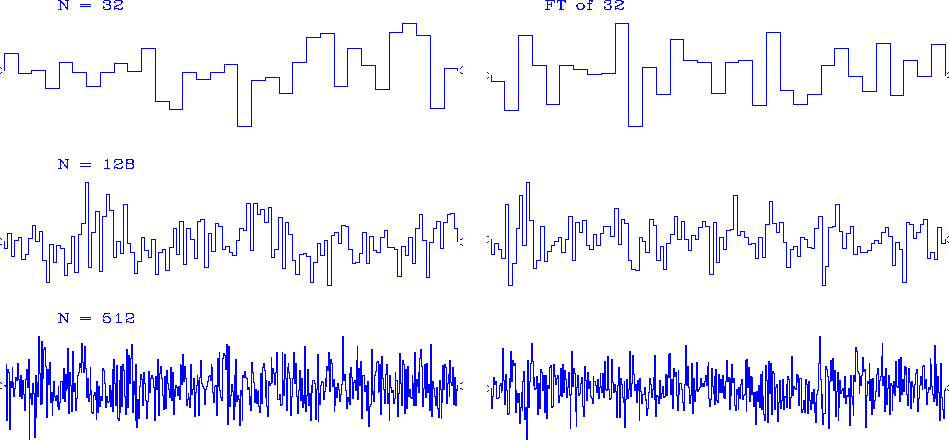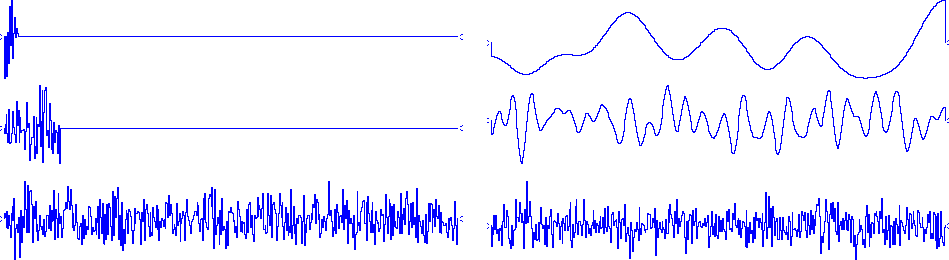Next: Bandlimited noise Up: Resolution and random signals Previous: Proof by way of

# FT OF RANDOM NUMBERS

Many real signals are complicated and barely comprehensible. In experimental work, we commonly transform such data. To better understand what this means, it will be worthwhile to examine signals made from random numbers.

Figure 3 shows discrete Fourier transforms of random numbers. The basic conclusion to be drawn from this figure is that transforms of random numbers look like more random numbers.nrand
Figure 3
Fourier cosine transforms of vectors containing random numbers. N is the number of components in the vector.

A random series containing all frequencies is called a ``white-noise" series, because the color white is made from roughly equal amounts of all colors. Any series made by independently chosen random numbers is said to be an ``independent" series. An independent series must be white, but a white series need not be independent.

Figure 4 shows Fourier transforms of random numbers surrounded by zeros (or zero padded).